Next: Complex Velocity Potential Up: Two-Dimensional Potential Flow Previous: Complex Functions

# Cauchy-Riemann Relations

We can define the derivative of a complex function in the same way that we define the derivative of a real function. In other words,(6.13)

However, we now have a problem. If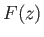is a well-behaved function (i.e., finite, single-valued, and differentiable) then it should not matter from which direction in the complex plane we approach the pointwhen taking the limit in Equation (6.13). There are, of course, many different possible approach directions, but if we look at a regular complex function,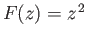(say), then(6.14)

is perfectly well-defined, and is, therefore, completely independent of the details of how the limit is taken in Equation (6.13).

The fact that Equation (6.13) has to give the same result, no matter from which direction we approach, means that there are some restrictions on the forms of the functions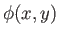and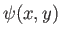in Equation (6.8). Suppose that we approachalong the real axis, so that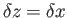. We obtain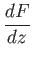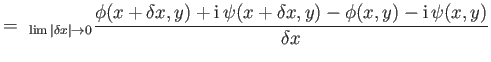(6.15)

Suppose that we now approachalong the imaginary axis, so that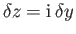. We get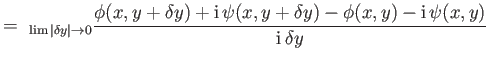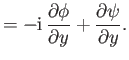(6.16)

But, ifis a well-behaved function then its derivative must be well-defined, which implies that the previous two expressions are equivalent. This requires that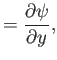(6.17)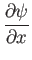(6.18)

These expressions are called the Cauchy-Riemann relations, and are, in fact, sufficient to ensure that all possible ways of taking the limit (6.13) give the same result (Riley 1974).

The Cauchy-Riemann relations can be combined to give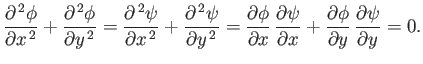(6.19)

In other words,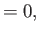(6.20)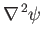(6.21)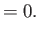(6.22)

It follows that the real and imaginary parts of a well-behaved function of the complex variable both satisfy Laplace's equation. Furthermore, the contours of these functions cross at right-angles.Next: Complex Velocity Potential Up: Two-Dimensional Potential Flow Previous: Complex Functions
Richard Fitzpatrick 2016-03-31